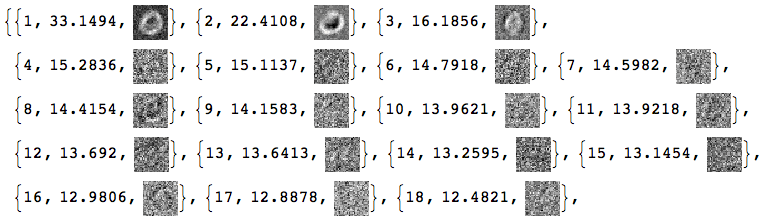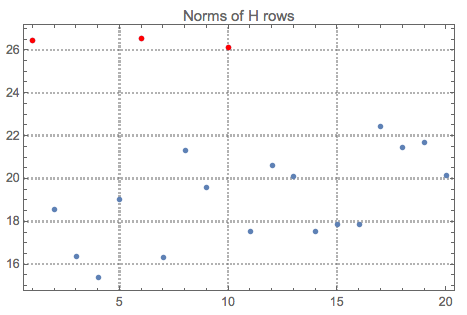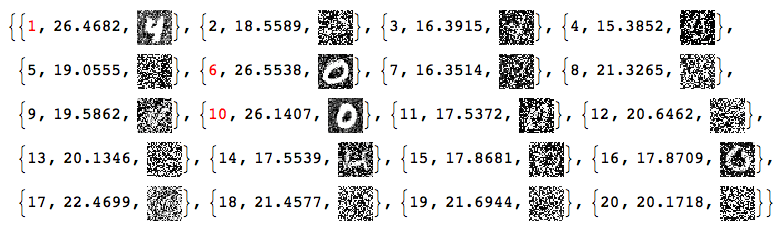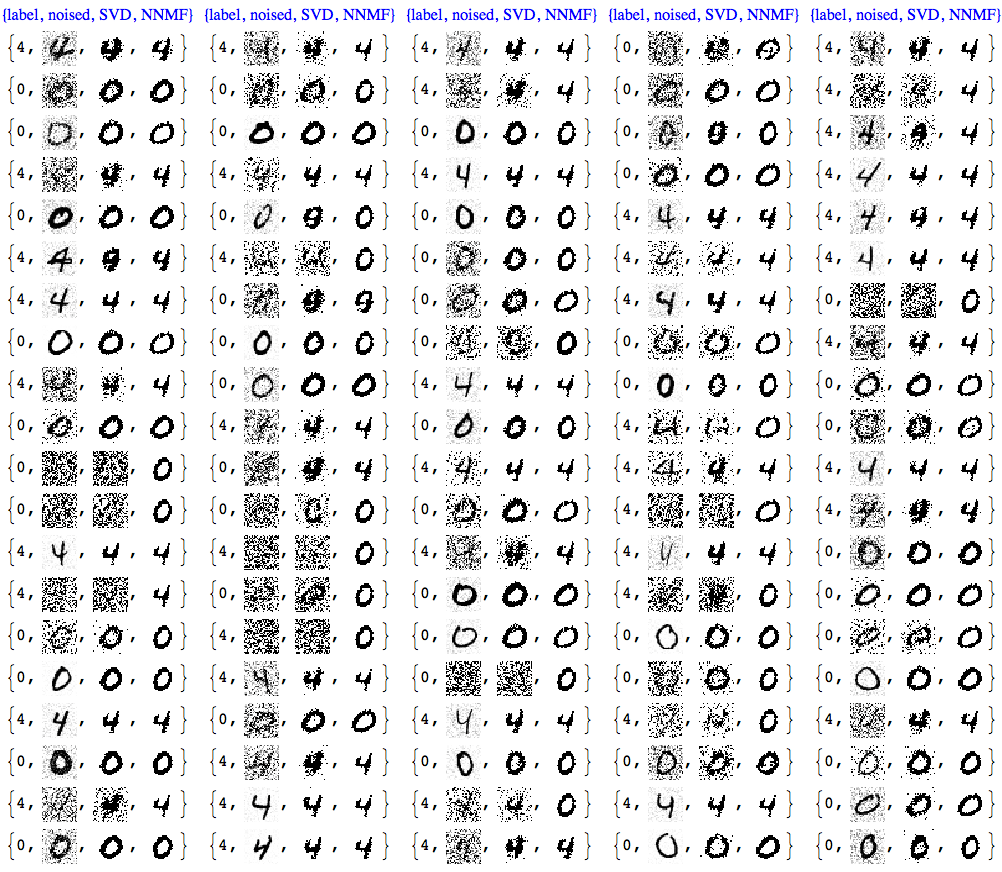# The Great conversation in USA presidential speeches

## Introduction

This document shows a way to chart in Mathematica / WL the evolution of topics in collections of texts. The making of this document (and related code) is primarily motivated by the fascinating concept of the Great Conversation, [Wk1, MA1]. In brief, all western civilization books are based ongreat ideas; if we find the great ideas each significant book is based on we can construct a time-line (spanning centuries) of the great conversation between the authors; see [MA1, MA2, MA3].

Instead of finding the great ideas in a text collection we extract topics statistically, using dimension reduction with Non-Negative Matrix Factorization (NNMF), [AAp3, AA1, AA2].

The presented computational results are based on the text collections of State of the Union speeches of USA presidents [D2]. The code in this document can be easily configured to use the much smaller text collection [D1] available online and in Mathematica/WL. (The collection [D1] is fairly small,documents; the collection [D2] is much larger,documents.)

The procedures (and code) described in this document, of course, work on other types of text collections. For example: movie reviews, podcasts, editorial articles of a magazine, etc.

A secondary objective of this document is to illustrate the use of the monadic programming pipeline as a Software design pattern, [AA3]. In order to make the code concise in this document I wrote the package MonadicLatentSemanticAnalysis.m, [AAp5]. Compare with the code given in [AA1].

The very first version of this document was written for the 2017 summer course “Data Science for the Humanities” at the University of Oxford, UK.

## Outline of the procedure applied

The procedure described in this document has the following steps.

1. Get a collection of documents with known dates of publishing.
• Or other types of tags associated with the documents.
2. Do preliminary analysis of the document collection.
• Number of documents; number of unique words.

• Number of words per document; number of documents per word.

• (Some of the statistics of this step are done easier after the Linear vector space representation step.)

3. Optionally perform Natural Language Processing (NLP) tasks.

1. Obtain or derive stop words.

2. Remove stop words from the texts.

3. Apply stemming to the words in the texts.

4. Linear vector space representation.

• This means that we represent the collection with a document-word matrix.

• Each unique word is a basis vector in that space.

• For each document the corresponding point in that space is derived from the number of appearances of document’s words.

5. Extract topics.

• In this document NNMF is used.

• In order to obtain better results with NNMF some experimentation and refinements of the topics search have to be done.

6. Map the documents over the extracted topics.

• The original matrix of the vector space representation is replaced with a matrix with columns representing topics (instead of words.)
7. Order the topics according to their presence across the years (or other related tags).
• This can be done with hierarchical clustering.

• Alternatively,

1. for a given topic find the weighted mean of the years of the documents that have that topic, and

2. order the topics according to those mean values.

8. Visualize the evolution of the documents according to their topics.

1. This can be done by simply finding the contingency matrix year vs topic.

2. For the president speeches we can use the president names for time-line temporal axis instead of years.

• Because the corresponding time intervals of president office occupation do not overlap.

Remark: Some of the functions used in this document combine several steps into one function call (with corresponding parameters.)

## Packages

This loads the packages [AAp1-AAp8]:

Import["https://raw.githubusercontent.com/antononcube/MathematicaForPrediction/master/MonadicProgramming/MonadicLatentSemanticAnalysis.m"];
Import["https://raw.githubusercontent.com/antononcube/MathematicaForPrediction/master/Misc/HeatmapPlot.m"];
Import["https://raw.githubusercontent.com/antononcube/MathematicaForPrediction/master/Misc/RSparseMatrix.m"];

(Note that some of the packages that are imported automatically by [AAp5].)

The functions of the central package in this document, [AAp5], have the prefix “LSAMon”. Here is a sample of those names:

Short@Names["LSAMon*"]

(* {"LSAMon", "LSAMonAddToContext", "LSAMonApplyTermWeightFunctions", <>, "LSAMonUnit", "LSAMonUnitQ", "LSAMonWhen"} *)

In this section we load a text collection from a specified source.

The text collection from “Presidential Nomination Acceptance Speeches”, [D1], is small and can be used for multiple code verifications and re-runnings. The “State of Union addresses of USA presidents” text collection from [D2] was converted to a Mathematica/WL object by Christopher Wolfram (and sent to me in a private communication.) The text collection [D2] provides far more interesting results (and they are shown below.)

If[True,
speeches = ResourceData[ResourceObject["Presidential Nomination Acceptance Speeches"]];
names = StringSplit[Normal[speeches[[All, "Person"]]][[All, 2]], "::"][[All, 1]],

(*ELSE*)
(*State of the union addresses provided by Christopher Wolfram. *)
Get["~/MathFiles/Digital humanities/Presidential speeches/speeches.mx"];
names = Normal[speeches[[All, "Name"]]];
];

dates = Normal[speeches[[All, "Date"]]];
texts = Normal[speeches[[All, "Text"]]];

Dimensions[speeches]

(* {2453, 4} *)

## Basic statistics for the texts

Using different contingency matrices we can derive basic statistical information about the document collection. (The document-word matrix is a contingency matrix.)

First we convert the text data in long-form:

docWordRecords =
Thread[{##}] &, {Range@Length@texts, names,
DateString[#, {"Year"}] & /@ dates,
DeleteStopwords@*TextWords /@ ToLowerCase[texts]}, 1];

Here is a sample of the rows of the long-form:

GridTableForm[RandomSample[docWordRecords, 6],
TableHeadings -> {"document index", "name", "year", "word"}]Here is a summary:

Multicolumn[
RecordsSummary[docWordRecords, {"document index", "name", "year", "word"}, "MaxTallies" -> 8], 4, Dividers -> All, Alignment -> Top]Using the long form we can compute the document-word matrix:

ctMat = CrossTabulate[docWordRecords[[All, {1, -1}]]];
MatrixPlot[Transpose@Sort@Map[# &, Transpose[ctMat@"XTABMatrix"]],
MaxPlotPoints -> 300, ImageSize -> 800,
AspectRatio -> 1/3]Here is the president-word matrix:

ctMat = CrossTabulate[docWordRecords[[All, {2, -1}]]];
MatrixPlot[Transpose@Sort@Map[# &, Transpose[ctMat@"XTABMatrix"]], MaxPlotPoints -> 300, ImageSize -> 800, AspectRatio -> 1/3]Here is an alternative way to compute text collection statistics through the document-word matrix computed within the monad LSAMon:

LSAMonUnit[texts]⟹LSAMonEchoTextCollectionStatistics[];## Procedure application

### Stop words

Here is one way to obtain stop words:

stopWords = Complement[DictionaryLookup["*"], DeleteStopwords[DictionaryLookup["*"]]];
Length[stopWords]
RandomSample[stopWords, 12]

(* 304 *)

(* {"has", "almost", "next", "WHO", "seeming", "together", "rather", "runners-up", "there's", "across", "cannot", "me"} *)

We can complete this list with additional stop words derived from the collection itself. (Not done here.)

### Linear vector space representation and dimension reduction

Remark: In the rest of the document we use “term” to mean “word” or “stemmed word”.

The following code makes a document-term matrix from the document collection, exaggerates the representations of the terms using “TF-IDF”, and then does topic extraction through dimension reduction. The dimension reduction is done with NNMF; see [AAp3, AA1, AA2].

SeedRandom

mObj =
LSAMonUnit[texts]⟹
LSAMonMakeDocumentTermMatrix[{}, stopWords]⟹
LSAMonApplyTermWeightFunctions[]⟹
LSAMonTopicExtraction[Max[5, Ceiling[Length[texts]/100]], 60, 12, "MaxSteps" -> 6, "PrintProfilingInfo" -> True];

This table shows the pipeline commands above with comments:#### Detailed description

The monad object mObj has a context of named values that is an Association with the following keys:

Keys[mObj⟹LSAMonTakeContext]

(* {"texts", "docTermMat", "terms", "wDocTermMat", "W", "H", "topicColumnPositions", "automaticTopicNames"} *)

Let us clarify the values by briefly describing the computational steps.

1. From texts we derive the document-term matrix, whereis the number of documents andis the number of terms.
• The terms are words or stemmed words.

• This is done with LSAMonMakeDocumentTermMatrix.

2. From docTermMat is derived the (weighted) matrix wDocTermMat using “TF-IDF”.

• This is done with LSAMonApplyTermWeightFunctions.
3. Using docTermMat we find the terms that are present in sufficiently large number of documents and their column indices are assigned to topicColumnPositions.

4. Matrix factorization.

1. Assign to,, where.

2. Compute using NNMF the factorization, where,, andis the number of topics.

3. The values for the keys “W, “H”, and “topicColumnPositions” are computed and assigned by LSAMonTopicExtraction.

5. From the top terms of each topic are derived automatic topic names and assigned to the key automaticTopicNames in the monad context.

• Also done by LSAMonTopicExtraction.

### Statistical thesaurus

At this point in the object mObj we have the factors of NNMF. Using those factors we can find a statistical thesaurus for a given set of words. The following code calculates such a thesaurus, and echoes it in a tabulated form.

queryWords = {"arms", "banking", "economy", "education", "freedom",
"tariff", "welfare", "disarmament", "health", "police"};

mObj⟹
LSAMonStatisticalThesaurus[queryWords, 12]⟹
LSAMonEchoStatisticalThesaurus[];By observing the thesaurus entries we can see that the words in each entry are semantically related.

Note, that the word “welfare” strongly associates with “[applause]”. The rest of the query words do not, which can be seen by examining larger thesaurus entries:

thRes =
mObj⟹
LSAMonStatisticalThesaurus[queryWords, 100]⟹
LSAMonTakeValue;
Cases[thRes, "[applause]", Infinity]

(* {"[applause]", "[applause]"} *)

The second “[applause]” associated word is “education”.

#### Detailed description

The statistical thesaurus is computed by using the NNMF’s right factor.

For a given term, its corresponding column inis found and the nearest neighbors of that column are found in the spaceusing Euclidean norm.

### Extracted topics

The topics are the rows of the right factorof the factorization obtained with NNMF .

Let us tabulate the topics found above with LSAMonTopicExtraction :

mObj⟹ LSAMonEchoTopicsTable["NumberOfTerms" -> 6, "MagnificationFactor" -> 0.8, Appearance -> "Horizontal"];### Map documents over the topics

The function LSAMonTopicsRepresentation finds the top outliers for each row of NNMF’s left factor. (The outliers are found using the package [AAp4].) The obtained list of indices gives the topic representation of the collection of texts.

Short@(mObj⟹LSAMonTopicsRepresentation[]⟹LSAMonTakeContext)["docTopicIndices"]

{{53}, {47, 53}, {25}, {46}, {44}, {15, 42}, {18}, <>, {30}, {33}, {7, 60}, {22, 25}, {12, 13, 25, 30, 49, 59}, {48, 57}, {14, 41}}

Further we can see that if the documents have tags associated with them — like author names or dates — we can make a contingency matrix of tags vs topics. (See [AAp8, AA4].) This is also done by the function LSAMonTopicsRepresentation that takes tags as an argument. If the tags argument is Automatic, then the tags are simply the document indices.

Here is a an example:

rsmat = mObj⟹LSAMonTopicsRepresentation[Automatic]⟹LSAMonTakeValue;
MatrixPlot[rsmat]Here is an example of calling the function LSAMonTopicsRepresentation with arbitrary tags.

rsmat = mObj⟹LSAMonTopicsRepresentation[DateString[#, "MonthName"] & /@ dates]⟹LSAMonTakeValue;
MatrixPlot[rsmat]Note that the matrix plots above are very close to the charting of the Great conversation that we are looking for. This can be made more obvious by observing the row names and columns names in the tabulation of the transposed matrix rsmat:

Magnify[#, 0.6] &@MatrixForm[Transpose[rsmat]]## Charting the great conversation

In this section we show several ways to chart the Great Conversation in the collection of speeches.

There are several possible ways to make the chart: using a time-line plot, using heat-map plot, and using appropriate tabulation (with MatrixForm or Grid).

In order to make the code in this section more concise the package RSparseMatrix.m, [AAp7, AA5], is used.

### Topic name to topic words

This command makes an Association between the topic names and the top topic words.

aTopicNameToTopicTable =
mObj⟹LSAMonTopicsTable["NumberOfTerms" -> 12]⟹LSAMonTakeValue];

Here is a sample:

Magnify[#, 0.7] &@ aTopicNameToTopicTable[[1 ;; 3]]### Time-line plot

This command makes a contingency matrix between the documents and the topics (as described above):

rsmat = ToRSparseMatrix[mObj⟹LSAMonTopicsRepresentation[Automatic]⟹LSAMonTakeValue]

This time-plot shows great conversation in the USA presidents state of union speeches:

TimelinePlot[
Association@
Tooltip[#2, aTopicNameToTopicTable[#2]] -> dates[[ToExpression@#1]] &,
Transpose[RSparseMatrixToTriplets[rsmat]]],
PlotTheme -> "Detailed", ImageSize -> 1000, AspectRatio -> 1/2, PlotLayout -> "Stacked"]The plot is too cluttered, so it is a good idea to investigate other visualizations.

### Topic vs president heatmap

We can use the USA president names instead of years in the Great Conversation chart because the USA presidents terms do not overlap.

This makes a contingency matrix presidents vs topics:

rsmat2 = ToRSparseMatrix[
mObj⟹LSAMonTopicsRepresentation[
names]⟹LSAMonTakeValue];

Here we compute the chronological order of the presidents based on the dates of their speeches:

nameToMeanYearRules =
Map[#[[1, 1]] -> Mean[N@#[[All, 2]]] &,
GatherBy[MapThread[List, {names, ToExpression[DateString[#, "Year"]] & /@ dates}], First]];
ordRowInds = Ordering[RowNames[rsmat2] /. nameToMeanYearRules];

This heat-map plot uses the (experimental) package HeatmapPlot.m, [AAp6]:

Block[{m = rsmat2[[ordRowInds, All]]},
HeatmapPlot[SparseArray[m], RowNames[m],
Thread[Tooltip[ColumnNames[m], aTopicNameToTopicTable /@ ColumnNames[m]]],
DistanceFunction -> {None, Sort}, ImageSize -> 1000,
AspectRatio -> 1/2]
]Note the value of the option DistanceFunction: there is not re-ordering of the rows and columns are reordered by sorting. Also, the topics on the horizontal names have tool-tips.

### Text data

[D1] Wolfram Data Repository, "Presidential Nomination Acceptance Speeches".

[D2] US Presidents, State of the Union Addresses, Trajectory, 2016. ‪ISBN‬1681240009, 9781681240008‬.

[D4] Gerhard Peters, "State of the Union Addresses and Messages", The American Presidency Project.

### Packages

[AAp1] Anton Antonov, MathematicaForPrediction utilities, (2014), MathematicaForPrediction at GitHub.

[AAp3] Anton Antonov, Implementation of the Non-Negative Matrix Factorization algorithm in Mathematica, (2013), MathematicaForPrediction at GitHub.

[AAp4] Anton Antonov, Implementation of one dimensional outlier identifying algorithms in Mathematica, (2013), MathematicaForPrediction at GitHub.

[AAp5] Anton Antonov, Monadic latent semantic analysis Mathematica package, (2017), MathematicaForPrediction at GitHub.

[AAp6] Anton Antonov, Heatmap plot Mathematica package, (2017), MathematicaForPrediction at GitHub.

[AAp7] Anton Antonov, RSparseMatrix Mathematica package, (2015), MathematicaForPrediction at GitHub.

[AAp8] Anton Antonov, Cross tabulation implementation in Mathematica, (2017), MathematicaForPrediction at GitHub.

### Books and articles

[AA1] Anton Antonov, "Topic and thesaurus extraction from a document collection", (2013), MathematicaForPrediction at GitHub.

[AA2] Anton Antonov, "Statistical thesaurus from NPR podcasts", (2013), MathematicaForPrediction at WordPress blog.

[AA3] Anton Antonov, "Monad code generation and extension", (2017), MathematicaForPrediction at GitHub.

[AA4] Anton Antonov, "Contingency tables creation examples", (2016), MathematicaForPrediction at WordPress blog.

[AA5] Anton Antonov, "RSparseMatrix for sparse matrices with named rows and columns", (2015), MathematicaForPrediction at WordPress blog.

[Wk1] Wikipedia entry, Great Conversation.

[MA1] Mortimer Adler, "The Great Conversation Revisited," in The Great Conversation: A Peoples Guide to Great Books of the Western World, Encyclopædia Britannica, Inc., Chicago,1990, p. 28.

[MA2] Mortimer Adler, "Great Ideas".

[MA3] Mortimer Adler, "How to Think About the Great Ideas: From the Great Books of Western Civilization", 2000, Open Court.

# Comparison of PCA and NNMF over image de-noising

This post compares two dimension reduction techniques Principal Component Analysis (PCA) / Singular Value Decomposition (SVD) and Non-Negative Matrix Factorization (NNMF) over a set of images with two different classes of signals (two digits below) produced by different generators and overlaid with different types of noise.

PCA/SVD produces somewhat good results, but NNMF often provides great results. The factors of NNMF allow interpretation of the basis vectors of the dimension reduction, PCA in general does not. This can be seen in the example below.

## Data

First let us get some images. I am going to use the MNIST dataset for clarity. (And because I experimented with similar data some time ago — see Classification of handwritten digits.).

MNISTdigits = ExampleData[{"MachineLearning", "MNIST"}, "TestData"];
{testImages, testImageLabels} =
Transpose[List @@@ RandomSample[Cases[MNISTdigits, HoldPattern[(im_ -> 0 | 4)]], 100]];
testImagesSee the breakdown of signal classes:

Tally[testImageLabels]
(* {{4, 48}, {0, 52}} *)

Verify the images have the same sizes:

Tally[ImageDimensions /@ testImages]
dims = %[[1, 1]]

Add different kinds of noise to the images:

noisyTestImages6 =
Table[ImageEffect[
testImages[[i]],
{RandomChoice[{"GaussianNoise", "PoissonNoise", "SaltPepperNoise"}], RandomReal}], {i, Length[testImages]}];Since the important values of the signals are 0 or close to 0 we negate the noisy images:

negNoisyTestImages6 = ImageAdjust@*ColorNegate /@ noisyTestImages6## Linear vector space representation

We unfold the images into vectors and stack them into a matrix:

noisyTestImagesMat = (Flatten@*ImageData) /@ negNoisyTestImages6;
Dimensions[noisyTestImagesMat]

(* {100, 784} *)


Here is centralized version of the matrix to be used with PCA/SVD:

cNoisyTestImagesMat =
Map[# - Mean[noisyTestImagesMat] &, noisyTestImagesMat];


(With NNMF we want to use the non-centralized one.)

Here confirm the values in those matrices:

Grid[{Histogram[Flatten[#], 40, PlotRange -> All,
ImageSize -> Medium] & /@ {noisyTestImagesMat,
cNoisyTestImagesMat}}]## SVD dimension reduction

For more details see the previous answers.

{U, S, V} = SingularValueDecomposition[cNoisyTestImagesMat, 100];
ListPlot[Diagonal[S], PlotRange -> All, PlotTheme -> "Detailed"]
dS = S;
Do[dS[[i, i]] = 0, {i, Range[10, Length[S], 1]}]
newMat = U.dS.Transpose[V];
denoisedImages =
Map[Image[Partition[# + Mean[noisyTestImagesMat], dims[]]] &, newMat];Here are how the new basis vectors look like:

Take[#, 50] &@
ImageAdjust@Image[Partition[Rescale[#3], dims[]]]} &, {Range[
Dimensions[V][]], Diagonal[S], Transpose[V]}]Basically, we cannot tell much from these SVD basis vectors images.

Here we load the packages for what is computed next:

Import["https://raw.githubusercontent.com/antononcube/MathematicaForPrediction/master/NonNegativeMatrixFactorization.m"]

Import["https://raw.githubusercontent.com/antononcube/MathematicaForPrediction/master/OutlierIdentifiers.m"]


The blog post “Statistical thesaurus from NPR podcasts” discusses an example application of NNMF and has links to documents explaining the theory behind the NNMF utilization.

The outlier detection package is described in a previous blog post “Outlier detection in a list of numbers”.

## NNMF

This command factorizes the image matrix into the product W H :

{W, H} = GDCLS[noisyTestImagesMat, 20, "MaxSteps" -> 200];
{W, H} = LeftNormalizeMatrixProduct[W, H];

Dimensions[W]
Dimensions[H]

(* {100, 20} *)
(* {20, 784} *)


The rows of H are interpreted as new basis vectors and the rows of W are the coordinates of the images in that new basis. Some appropriate normalization was also done for that interpretation. Note that we are using the non-normalized image matrix.

Let us see the norms of $H$ and mark the top outliers:

norms = Norm /@ H;
ListPlot[norms, PlotRange -> All, PlotLabel -> "Norms of H rows",
PlotTheme -> "Detailed"] //
ColorPlotOutliers[TopOutliers@*HampelIdentifierParameters]
OutlierPosition[norms, TopOutliers@*HampelIdentifierParameters]
OutlierPosition[norms, TopOutliers@*SPLUSQuartileIdentifierParameters]Here is the interpretation of the new basis vectors (the outliers are marked in red):

MapIndexed[{#2[], Norm[#], Image[Partition[#, dims[]]]} &, H] /. (# -> Style[#, Red] & /@
OutlierPosition[norms, TopOutliers@*HampelIdentifierParameters])Using only the outliers of $H$ let us reconstruct the image matrix and the de-noised images:

pos = {1, 6, 10}
dHN = Total[norms]/Total[norms[[pos]]]*
DiagonalMatrix[
ReplacePart[ConstantArray[0, Length[norms]],
Map[List, pos] -> 1]];
newMatNNMF = W.dHN.H;
denoisedImagesNNMF =
Map[Image[Partition[#, dims[]]] &, newMatNNMF];


Often we cannot just rely on outlier detection and have to hand pick the basis for reconstruction. (Especially when we have more than one classes of signals.)

## Comparison

At this point we can plot all images together for comparison:

imgRows =
Transpose[{testImages, noisyTestImages6,
With[{ncol = 5},
Grid[Prepend[Partition[imgRows, ncol],
Style[#, Blue, FontFamily -> "Times"] & /@
Table[{"original", "noised", "SVD", "NNMF"}, ncol]]]]We can see that NNMF produces cleaner images. This can be also observed/confirmed using threshold binarization — the NNMF images are much cleaner.

imgRows =
With[{th = 0.5},
MapThread[{#1, #2, Binarize[#3, th],
Binarize[#4, th]} &, {testImageLabels, noisyTestImages6,
With[{ncol = 5},
Grid[Prepend[Partition[imgRows, ncol],
Style[#, Blue, FontFamily -> "Times"] & /@
Table[{"label", "noised", "SVD", "NNMF"}, ncol]]]]Usually with NNMF in order to get good results we have to do more that one iteration of the whole factorization and reconstruction process. And of course NNMF is much slower. Nevertheless, we can see clear advantages of NNMF’s interpretability and leveraging it.

## Gallery with other experiments

In those experiments I had to hand pick the NNMF basis used for the reconstruction. Using outlier detection without supervision would not produce good results most of the time.

## Further comparison with Classify

We can further compare the de-noising results by building signal (digit) classifiers and running them over the de-noised images.

For such a classifier we have to decide:

1. do we train only with images of the two signal classes or over a larger set of signals;
2. how many signals we train with;
3. with what method the classifiers are built.

Below I use the default method of Classify with all digit images in MNIST that are not in the noised images set. The classifier is run with the de-noising traced these 0-4 images:

Get the images:

{trainImages, trainImageLabels} = Transpose[List @@@ Cases[MNISTdigits, HoldPattern[(im_ -> _)]]];
pred = Map[! MemberQ[testImages, #] &, trainImages];
trainImages = Pick[trainImages, pred];
trainImageLabels = Pick[trainImageLabels, pred];
Tally[trainImageLabels]

(* {{0, 934}, {1, 1135}, {2, 1032}, {3, 1010}, {4, 928}, {5, 892}, {6, 958}, {7, 1028}, {8, 974}, {9, 1009}} *)
 

Build the classifier:

digitCF = Classify[trainImages -> trainImageLabels]

 

Compute classifier measurements for the original, the PCA de-noised, and NNMF de-noised images:

origCM = ClassifierMeasurements[digitCF, Thread[(testImages) -> testImageLabels]]

pcaCM = ClassifierMeasurements[digitCF,
Thread[(Binarize[#, 0.55] &@*ImageAdjust@*ColorNegate /@ denoisedImages) -> testImageLabels]]

nnmfCM = ClassifierMeasurements[digitCF,
Thread[(Binarize[#, 0.55] &@*ImageAdjust@*ColorNegate /@  denoisedImagesNNMF) -> testImageLabels]]
 

Tabulate the classification measurements results:

Grid[{{"Original", "PCA", "NNMF"},
{Row[{"Accuracy:", origCM["Accuracy"]}], Row[{"Accuracy:", pcaCM["Accuracy"]}], Row[{"Accuracy:", nnmfCM["Accuracy"]}]},
{origCM["ConfusionMatrixPlot"], pcaCM["ConfusionMatrixPlot"], nnmfCM["ConfusionMatrixPlot"]}},
Dividers -> {None, {True, True, False}}]# Finding outliers in 2D and 3D numerical data

## Introduction

This blog post describes a method of finding outliers in 2D and 3D data using Quantile Regression Envelopes discussed in previous blog posts: “Directional quantile envelopes”, “Directional quantile envelopes in 3D”.

## Data

In order to provide a good example of the method application it would be better to use “real life” data. I found this one: “UCI Online Retail Data Set”. That dataset has columns for online purchase transactions (quantity and price).

(*data=Import["http://archive.ics.uci.edu/ml/machine-learning-databases/00352/Online%20Retail.xlsx","XLSX"];*)


so I downloaded the XLSX file and saved it into a CSV file, then imported it:

data = Import["~/Datasets/UCI Online Retail Data Set/Online Retail.csv",
"IgnoreEmptyLines" -> True, "HeaderLines" -> 0];
columnNames = data[];
data = Rest[data];


Here are the dimensions of the dataset (seems “realistically” large):

Dimensions[data]
(* {65499, 8} *)


Here is a summary of the quantative columns:

Grid[{RecordsSummary[N@data[[All, {4, 6}]], columnNames[[{4, 6}]]]}, Dividers -> All]### Adding a “discount” column

Since we have only two quantative columns in the data let us add a third one, and make it have 3 outliers.

dvec = RandomReal[SkewNormalDistribution[1, 2, 4], Dimensions[data][]];
Block[{inds = RandomSample[Range[Length[dvec]], 3]},
dvec[[inds]] = 10*dvec[[inds]]];
testData = MapThread[Append, {N@data[[All, {4, 6}]], dvec}];


Here is the summary:

Grid[{RecordsSummary[testData]}, Dividers -> All]### Standardizing

It is a good idea to standardize the data. This is not necessary for the outlier finding procedure used below, but it makes the data more convenient for visualization or other exploration.

sTestData = Transpose[Standardize /@ Transpose[N@testData]];

Because of the outliers plotting the data might produce uninformative plots. We can use logarithms of the point coordinates and for that we have to shift the standardized data to be positive. This is done with this command:

Block[{offset = -2 (Min /@ Transpose[sTestData])},
sTestData = Map[# + offset &, sTestData]];


Let use show the standardized data summary and visualize:

Grid[{RecordsSummary[sTestData]}, Dividers -> All]opts = {PlotRange -> All, ImageSize -> Medium,
PlotTheme -> "Detailed"}; Grid[{{ListPointPlot3D[sTestData, opts],
ListPointPlot3D[Log10@sTestData, opts]}}]## Using Quantile Regression envelopes to find outliers

We are going to find the outliers by computing envelopes around the dataset points that contain almost all points (e.g. 99.7% of them).

The finding of directional quantile envelopes in 2D and 3D is explained in these blog posts:

I am a big fan of Quantile Regression (QR) and I have implemented a collection of functions and applications of QR. See these blog posts.

This command imports the package QuantileRegression.m :

Import["https://raw.githubusercontent.com/antononcube/MathematicaForPrediction/master/QuantileRegression.m"]


Here is an example of an envelope found using directional quantiles over a small sample of the points:

Block[{testData = RandomSample[sTestData, 2000], qreg},
qreg = QuantileEnvelopeRegion[testData, 0.997, 10];
Show[{Graphics3D[{Red, Point[testData]}, Axes -> True],
BoundaryDiscretizeRegion[qreg]}]
]The outlier points (in red) are outside of the envelope.

The example (and plot) above are just for illustration purposes. We calculate a quantile evelope region using all points.

Block[{testData = sTestData},
qreg = QuantileEnvelopeRegion[testData, 0.9997, 10];
]


(The function QuantileEnvelopeRegion works also for 2D. For 2D nothing else changes in the steps below except using 2D graphics.)

This command makes a function to test does a point belong to the found envelope region or not:

rmFunc = RegionMember[qreg];


This calculates the membership predicates for all points:

AbsoluteTiming[
pred = rmFunc /@ sTestData;
]

(* {15.6485, Null} *)


And we can see the membership breakdown:

Tally[pred]
(* {{True, 65402}, {False, 97}} *)


and visualize it (using Pick and taking logarithms):

Graphics3D[{Gray, Point[Log10@Pick[sTestData, pred]], Red,
Point[Log10@Pick[sTestData, Not /@ pred]]}, Axes -> True]The plot above contains both top and bottom outliers (in red). If we are intereseted only in the top outliers we can find these thresholds:

topThresholds = Quantile[#, 0.95] & /@ Transpose[testData]
(* {25, 10.95, 4.89433} *)


and use them to select the top outliers:

Select[Pick[testData, Not /@ pred],
Total[Thread[# > topThresholds] /. {True -> 1, False -> 0}] > 1 &]

(* {{1, 836.14, 6.48564}, {1, 16.13, 8.71455}, {5, 25.49, 8.47351}, {-1, 1126, 5.25211}, {1000, 0, 5.4212}, {1, 15.79, 9.14674}, {-1, 544.4, 6.86266}} *)


Note that the quantile regression envelope and the membership predicates were computed over the standardized data, and the predicates were used to retrieve the outliers of the original data.